The Rational Root Theorem
 Home > Lessons > Rational Root Theorem Search | Updated September 16th, 2019
Introduction
This lesson will inform you how to find all of the rational roots (zeros) of a polynomial. Here are the sections within this lesson:

 Roots versus Zeros The terms roots and zeros get tossed around as if they are interchangeable. They do indicate two different mathematical ideas.     Zeros are places along the x-axis where a polynomial intersects it. Those locations are called x-intercepts.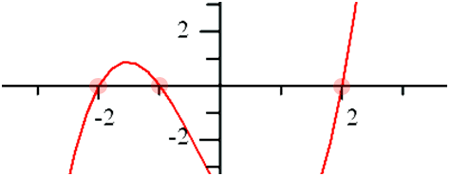The graph of the polynomial above has zeros at -2, -1, and 2.     Roots of a polynomial can be found by setting a polynomial equal to zero. The solutions are called roots.     Take a look at the polynomial function f(x) below, which is in factored form. To find its roots, we need only set the function equal to zero and solve.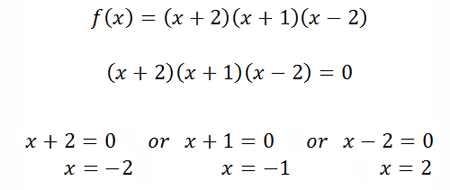We can see that f(x) has the roots -2,-1, and 2.     Now you may be thinking, “Aren’t the solutions to the equations (called ‘roots’) also the x-intercepts of the polynomial?” That would be a fantastic question to have and to ask. The answer to the question is ‘yes.’ This is why the terms roots and zeros are often times used interchangeably. Roots and zeros, although have different meaning, have the same values. The Rational Root Theorem Given a polynomial, there is a process we can follow to find all of its possible rational roots. This process is defined within the Rational Root Theorem, which states:     All the possible rational roots of a polynomial can be represented as p/q, such that…Watch our video on this topic.ideo: The Rational Root Theorem Finding Possible Rational Roots of a Polynomial Let us examine a polynomial, g(x), to understand and practice the Rational Root Theorem.     Here is g(x).We can see g(x) is written in a special manner, called descending form. This means the term with the highest degree is written first and the term with the next highest term is written, and so on until we reach the constant term.     So, the constant term is 6 and the leading coefficient is 3.     First, we find all the possible factors of the constant term. We ask ourselves, “What numbers divide into 6 and have no remainder?” Here are those numbers:However, we also have to consider positive and negative values that divide into 6 evenly, which would be p.Second,we have to find all of the possible factors of the leading coefficient.We ask ourselves, “What numbers divide into 3 and have no remainder?” Those numbers are…Finally, we have to determine the ratio of all the possible factors of the constant term over the possible factors of the leading term, like so:If all of the factors of the constant are divided by positive or negative 1, we get…If all of the factors of the constant are divided by positive or negative 3, we get……which is the same as…Now, let’s put all of these possible factors together and not repeat factors. These are all of the possible rational roots of g(x).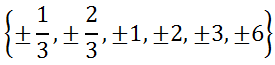Watch our video on this topic.ideo: The Rational Root Theorem Finding Actual Rational Roots of a Polynomial The only way to tell if a possible roots is an actual roots is to test it. We can either use synthetic division or the remainder theorem. The polynomial from the previous section, g(x), will be our focus.Synthetic division is covered to great deal in another lesson (see Operations on Polynomials). Nevertheless, here is some work shown using synthetic division. Remember, the objective is to find a value with synthetic division that produces a zero remainder.     Let us look at -3 as a possible root. This will also be a test to see if (x + 3) is a factor of the polynomial.-3 is not a root because it does not have a zero remainder. Let’s try 3 or (x - 3).We see that 3 is a root because (x - 3) produces a zero remainder. With the remaining polynomial, let’s try -2.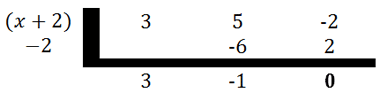Since the remainder is zero, this means (x+2) is a factor and -2 is a zero. The remaining polynomial is (3x - 1).     To determine the last zero of g(x), set the remaining polynomial equal to zero and solve for the x-value.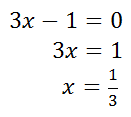So, the roots for g(x) are…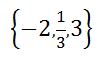If we write g(x) as a polynomial function in factored form,we get…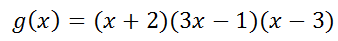Watch our video on this topic.ideo: The Rational Root Theorem Instructional Videos View our instructional video related to The Rational Root Theorem.ideo: The Rational Root Theorem Related Lessons Try these lessons, which are closely related to the lesson above.esson: Functionsesson: Polynomials, Factors, and Zerosesson: Translationsesson: Complex Numbers